# Introduction to Python – Part 2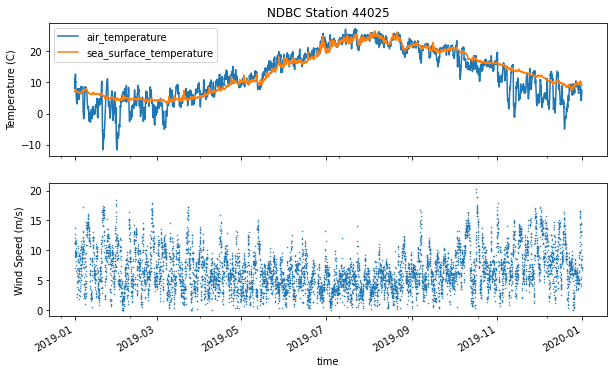Teaching students how to visualize ocean data is a challenge. But before you get into cognitive theory, choosing colors, or the the principles of (good) visualization design, you really just need to get your students’ feet wet plotting some data.

This summer, as part our Virtual REU 2-week mini-workshop, we challenged students to work in groups to find interesting stories in the NDBC dataset. But before setting them off on their mini-research projects, we spent three sessions introducing students to python, NDBC data, and basic plotting.

As described in Part 1, in our first session we introduced students to python and the Google Colab environment. In the second session, students worked in groups to practice these basics, while making quick plots of the NDBC buoy data. Finally, in our third session, we demonstrated some python plotting basics using following notebook. If you’re curious, here are a few slides I also used as 30-second introduction to Data Vis.

### Data Visualization Basics

The notebook below features some, but by no means all, of the ways you can create and customize plots in python, focusing primarily on timeseries plots.

The notebook briefly touches on customizing marks and lines, changing axes labels and limits, adding legends, and using subplots. Almost as an afterthought, I included a brief mention of scatterplots, histograms, and box plots, because those plots are so easy to make in python with just one line of code – assuming you know what you’re doing. I felt it was good to introduce students to a few additional plot types that are relevant to time series datasets, while also demonstrating the power of the tool to encourage students to explore more in the future.

It turns out, even simple isn’t that simple. As this was my first time teaching this skill to novice learners, I discovered quite a few hangups students can run into. Here are just a few:

• xarray vs. pandas – Both of these libraries are wonderful. While pandas is designed for “tabular” datasets, like those you find in Excel, xarray is a bit more complicated as it is designed to support multi-dimensional datasets. If your data is simple, like a CSV file with a timeseries or discrete data points, pandas is all you need. It has a far simpler data-model, and is a bit more intuitive for data analysis or visualization. Unfortunately, the NDBC dataset is served over THREDDS, which requires using the xarray library to access it. You’ll see in the notebook I ran through some hoops to convert the xarray Dataset to a pandas Dataframe, in the hopes that it might make the rest of the notebook easier to follow. But this was definitely not easy to get across to students at the very beginning. If you can, I’d suggest sticking with pandas-friendly datasets to start.
• Internal plotting functions – Both xarray and pandas have internal plotting methods that allow you to quickly create plots, without having to call matplotlib explicitly, e.g. with plt.plot(). These methods are also pretty “smart” in that they will label and title your graphs using information in your data, using column names and units if available. That’s a great feature, if you know how the black boxes work, but it’s yet another confusing point for students. For example, the syntax for `plt.plot()`, `xr.Dataset.plot()` and `pd.Dataframe.plot()` can differ, depending on what you’re trying to do. While I love the internal methods, in the future, for a basic intro to plotting, I think I’ll start with matplotlib, and have students create graphs/axes/labels manually, allowing them to discover the benefits of the internal methods later.
• plot vs. scatterplot – This one is on me. For some reason, in this notebook, when I wanted to create a scatter plot, I used `plt.plot(x, y, linestyle='', marker='.')` instead of just calling `plt.scatter()`. While it’s good to remind students that there are often many way to solve a problem, especially in python, it’s also good to keep things simple to start.
• datetime – Dates and times are the bane of every programmer. Python has a lot of great tools for working with dates (some might argue too many), but you still have to figure them out. Sometimes that beautiful looking date-time array in your Dataframe isn’t actually datetime.datetime() friendly, but rather just an array of strings. To a human it looks the same. But to the computer, they’re totally different. And that takes some time to learn. That said, I have no idea how to teach this quickly or well, other than suggesting you make sure your datasets and examples are clear.
• NDBC Data – Finally, just a note on NDBC… I absolutely love this dataset, because the variables are familiar to students, it is (relatively) easy to access, and it has a global coverage allowing students to explore a variety of questions. However, like every other data portal out there, you can get confused the datasets that are actually available. On the homepage, NDBC lists over 1,400 stations, but only about 150 are meteorological buoys in the ocean. We had quite a few students interested in data from estuarine stations, tide gauges, and the TAO array. Sadly, not all of that data was as easy to access as the blue water buoys.

If I have a chance to teach an introduction to data visualization course in the future (ideally with more time), I hope to figure out some new approaches to these challenges. And if you have any ideas, I’d love to hear them too!

But in the end, I think students in our REU really appreciated having this notebook, with its collection of examples to refer to, as they worked on their own plots.

In fact, I hadn’t originally intended to include the two axes example in the notebook, but it was something students really wanted to know how to do. (In the data vis community, many have made arguments against duel axes charts, but they’re pretty common in oceanography.)

At any rate, if you are working on your own ocean/python/datavis introduction, I hope you find this notebook helpful!

This post is part of our 2020 Summer REU Intro to Python series. See also Part 1, Part 3, and Part 4.

# Activity 2 - Data Visualization¶

2020 Data Labs REU

Written by Sage Lichtenwalner, Rutgers University, June 12, 2020

In this notebook we will cover some of basics of plotting in python, primarily using the matplotlib library. We've actually already used this library, as it is built into the pandas and xarray libraries to provide quick plotting capabilities. But if we want to customize our charts, it's often better to create them directly using matplotlib function calls.

The examples today will continue to use the mooring timeseries data available from NDBC in order to demonstrate timeseries, scatterplots, histograms and box plots.

For an example of other graph types commonly seen in oceanography, including profiles and TS diagrams, check out Bonus Activity 4, which demonstrates how to load and plot profile data from the ARGO drifter network.

In :
```# Notebook setup
import xarray as xr
!pip install netcdf4

import matplotlib.pyplot as plt
```
```Requirement already satisfied: netcdf4 in /usr/local/lib/python3.6/dist-packages (1.5.3)
Requirement already satisfied: numpy>=1.7 in /usr/local/lib/python3.6/dist-packages (from netcdf4) (1.18.5)
Requirement already satisfied: cftime in /usr/local/lib/python3.6/dist-packages (from netcdf4) (1.1.3)
```

# NDBC Timeseries¶

Following our example from yesterday, let's load some timeseries data from an NDBC mooring. We will use this dataset to show how to customize your plot.

In :
```# Open dataset
ds = xr.open_dataset('https://dods.ndbc.noaa.gov/thredds/dodsC/data/stdmet/44025/44025.ncml')

# Subset the dataset to 1 year
ds = ds.sel(time=slice('2019-01-01','2020-01-01'))
```

## Convert Xarray Dataset to Pandas Dataframe¶

Yesterday we used the power of Xarray to load our NDBC dataset directly from a Thredds server. Xarray is great, especially when dealing with 3D or 4D datasets. But it can overcomplicate things. For example, our NDBC dataset actually loads with 3 dimensions (time, latitude and longitude), but we only need 1 (time).

Here are a few example plotting calls. Can you tell what's different in the output for each?

In :
```# Built in xarray plotting
# ds.sea_surface_temperature.plot();

# Plot using matplotlib - This won't work
# plt.plot(ds.sea_surface_temperature);

# Plot using matplotlib - This will, but the units are wrong
# plt.plot(ds.sea_surface_temperature.squeeze())

# Plot using matplotlib - Correctly plotted with time
# plt.plot(ds.time,ds.sea_surface_temperature.squeeze());
```

To simply things, we can convert our Xarray Dataset to a Pandas Dataframe, which will give use something like a spreadsheet of columns for each variable, and rows for each measurement time.

Here's how easy it is to convert.

In :
```# Convert to Pandas Dataframe
df = ds.to_dataframe()
```
Out:
wind_dir wind_spd gust wave_height dominant_wpd average_wpd mean_wave_dir air_pressure air_temperature sea_surface_temperature dewpt_temperature visibility water_level
latitude longitude time
40.250999 -73.164001 2019-01-01 00:50:00 135.0 11.3 14.1 1.56 00:00:05.559999 00:00:04.710000 153.0 1018.400024 8.1 7.1 NaN NaN NaN
2019-01-01 01:50:00 127.0 11.2 14.0 1.92 00:00:05.880000 00:00:05.090000 135.0 1016.599976 8.3 7.1 NaN NaN NaN
2019-01-01 02:50:00 137.0 12.7 15.3 2.23 00:00:06.250000 00:00:05.219999 143.0 1013.599976 8.7 7.2 NaN NaN NaN
2019-01-01 03:50:00 150.0 13.7 16.9 2.52 00:00:06.670000 00:00:05.489999 148.0 1010.700012 9.5 7.2 NaN NaN NaN
2019-01-01 04:50:00 168.0 9.5 11.2 2.77 00:00:07.139999 00:00:05.750000 141.0 1010.000000 10.2 7.2 NaN NaN NaN

Unfortunately, there's still a bit of complexity here because of the multi-dimensional index. If we try to plot this now, we get some crazy labels.

In :
```df.sea_surface_temperature.plot();
```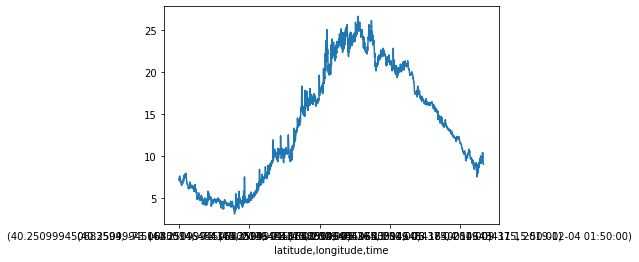Here's how we can properly convert this Dataset to a Dataframe.

In :
```# Convert to Pandas Dataframe
df = ds.to_dataframe().reset_index().set_index('time')
```
Out:
latitude longitude wind_dir wind_spd gust wave_height dominant_wpd average_wpd mean_wave_dir air_pressure air_temperature sea_surface_temperature dewpt_temperature visibility water_level
time
2019-01-01 00:50:00 40.250999 -73.164001 135.0 11.3 14.1 1.56 00:00:05.559999 00:00:04.710000 153.0 1018.400024 8.1 7.1 NaN NaN NaN
2019-01-01 01:50:00 40.250999 -73.164001 127.0 11.2 14.0 1.92 00:00:05.880000 00:00:05.090000 135.0 1016.599976 8.3 7.1 NaN NaN NaN
2019-01-01 02:50:00 40.250999 -73.164001 137.0 12.7 15.3 2.23 00:00:06.250000 00:00:05.219999 143.0 1013.599976 8.7 7.2 NaN NaN NaN
2019-01-01 03:50:00 40.250999 -73.164001 150.0 13.7 16.9 2.52 00:00:06.670000 00:00:05.489999 148.0 1010.700012 9.5 7.2 NaN NaN NaN
2019-01-01 04:50:00 40.250999 -73.164001 168.0 9.5 11.2 2.77 00:00:07.139999 00:00:05.750000 141.0 1010.000000 10.2 7.2 NaN NaN NaN
In :
```# Yes, even Pandas has built in plotting
df.sea_surface_temperature.plot();
```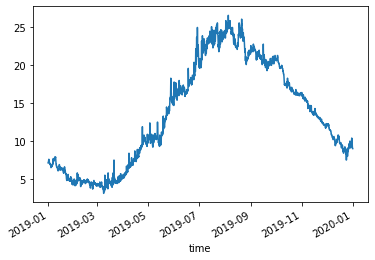And now we're off to the races (again).

### Exporting Data¶

One quick aside... Pandas also allows you to quickly export your data, which you can use to load the dataset into another program like Excel. Here's a quick example.

In :
```df[['air_temperature','sea_surface_temperature','wind_spd']].to_csv('44025data.csv')
```

## Customizing Timeseries Plots¶

Matplotlib provides quite a few ways to customize your plot.

### Customizing Lines¶

Here are some of the more common parameters you will typically use when creating your plot.

• linewidth - For example 0.5, 1, 2...
• linestyle - For example '-','--', or ':' or other basic or advanced styles
• color
• marker
• label - The name of the line, used in a legend (see the next section)

For reference and inspiration, you can also check out the Matplotlib Gallery.

In :
```# Line Example
plt.plot(df.index,df.sea_surface_temperature, color='red', linewidth=3)
```
Out:
`[<matplotlib.lines.Line2D at 0x7fada48629b0>]`In :
```# Custom Markers Example
plt.plot(df.index,df.sea_surface_temperature, color='red', linestyle='',
marker='d', markerfacecolor='b', markeredgecolor='g', markersize=5)
```
Out:
`[<matplotlib.lines.Line2D at 0x7fada47ef320>]`In :
```# Your Turn - Create a graph of air temperature using blue dots
```

### Customizing the Axis¶

• Axis Title: `plt.title('Title')`
• Legend: `plt.legend()`
• Axes Labels: `plt.xlabel('Time')` or `plt.ylabel('Temperature')`
• Axes Limits: `plt.ylim([-5,5])`
In :
```# Incomplete Example
plt.plot(df.index,df.air_temperature, color='red')
plt.plot(df.index,df.sea_surface_temperature, color='blue', label='Sea Surface Temp')
plt.legend();
```In :
```# Your Turn - Fix the legend, and add a title and y label to the above plot.
```

### Customizing Time Axes Limits¶

There are a few ways you can change the x-axis limits when you are working with timeplots. By default, plots will show the full range of data, with a little bit of padding on each side.

To plot just the full Full Time Range of data, you can use `plt.xlim(df.index.min(),df.index.max())`

To plot a Specific Time Range, you can use `plt.xlim(datetime.date(2017,4,1),datetime.date(2019,10,1))` Note you will need to run `import datetime` first for this command to run.

When you customize date limits you may also need to rotate your tick labels to prevent them from overlapping. One solution that might work is `plt.xticks(rotation=45)`

In :
```# Your Turn - Try changing the y and/or x limits for the above plot
```

We can create a figure with multiple plots using the subplots feature.

And we can save a figure to a file using `fig.savefig()`

In :
```# Subplot example
fig, (ax1,ax2) = plt.subplots(2,1, sharex=True, figsize=(10,6))

df.air_temperature.plot(ax=ax1)
df.sea_surface_temperature.plot(ax=ax1)
df.wind_spd.plot(ax=ax2, marker='.',linestyle='',markersize=1)

ax1.legend()
ax1.set_ylabel('Temperature (C)')
ax2.set_ylabel('Wind Speed (m/s)')
ax1.set_title('NDBC Station 44025');

# Save the figure to a file
fig.savefig('44025_example.png')
```In :
```# Your Turn - Recreate the above plot with a 3rd or 4th subplot using other variables
```

## Scatterplot¶

When two variables are plotted against each other, this is typically called a scatterplot. They are really no different than the plots we crated above. We just need to pick two variables, and use a marker instead of a line.

In :
```# One way - Using a modified plot() call
plt.plot(df.sea_surface_temperature,df.air_temperature, linestyle='', marker='.', markersize=3)

# Another (better) way - Using scatter()
# plt.scatter(df.sea_surface_temperature, df.air_temperature, s=3)

plt.xlabel('Sea Surface Temperature (C)')
plt.ylabel('Air Temperature (C)')
plt.title('NDBC Station 44025 from 1/1/2019 to 1/1/2020');
```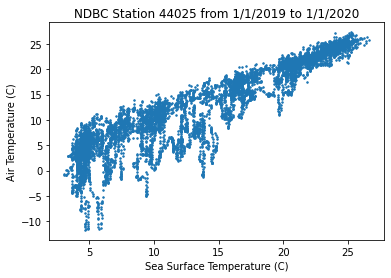In :
```# Your Turn - Create a scatterplot of winds vs. waves
```

One of the big advantages of the `.scatter()` function, is that you can also color and size the dots based on a variable, rather than having them all be the same.

In :
```# Your Turn - Now try coloring it using temperature or time
```

## Histogram¶

We can easily create histograms of a single variable. Use the `bins` parameter to increase or decrease the number of data bins.

In :
```# We can also easily create histograms
df['sea_surface_temperature'].hist(bins=50);
```In :
```# Your Turn - Create a histogram of another variable
```

## Box Plots¶

You can also create box plots of your data rather easily. You could also create violin plots.

In :
```# And boxplots
df[['sea_surface_temperature','air_temperature','wind_spd']].plot.box(vert=False);
```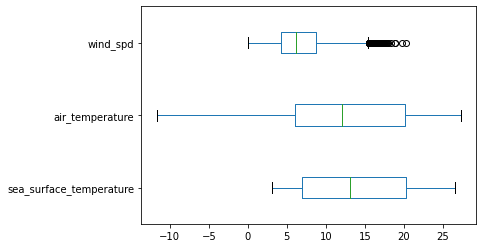## Bar Plots¶

Bar plots are a very common data visualization, but not typically used with this kind of dataset.

That said, a bar plot could be used to show monthly averages (more on how to calculate them tomorrow) or anomalies.

Here's a quick (albeit crude) example that shows the monthly averages for the dataset we've been working with.

In :
```df.sea_surface_temperature.resample('M').mean().plot.bar();
```Obviously, we'd need to work on the date labels, but hopefully this gives you a general idea.

## Two Axes on the same Plot¶

In addition to using subplots, some scientists like to plot two variables on the same graph. For example, you can create two y-axes using the left and right sides. Using 2 x-axes is also common with CTD profile plots.

Personally I'm not a huge fan of this, but it can be effective for some datasets and audiences, like your fellow scientists who are used to this typ of graph. Just don't try to plot more than 2 axes together, that's just heresy 😉

The following example uses 2 y-axes to plot both Water Temperature and Dissolved Oxygen from an estuarine site near Atlantic City. This was adapted from this example.

Also, noticed how we can load, subset and convert from xarray to pandas all in one line. This "chaining" of commands, is one of the great features of Python.

In :
```# Load a JCNERR Estuarine Station from NDBC
nerr = xr.open_dataset('https://dods.ndbc.noaa.gov/thredds/dodsC/data/ocean/jctn4/jctn4o9999.nc')
nerr = nerr.sel(time=slice('2019-06-01','2020-06-01')).to_dataframe().reset_index().set_index('time')
```
In :
```# A graph with 2 Y-axes
fig, ax1 = plt.subplots()
ax2 = ax1.twinx() # Create a second axes that shares the same x-axis

color = 'tab:red'
ax1.plot(nerr.index, nerr.water_temperature, color=color)
ax1.set_ylabel('Water Temperature (C)', color=color)
ax1.tick_params(axis='y', labelcolor=color)

color = 'tab:blue'
ax2.plot(nerr.index, nerr.o2_saturation, color=color)
ax2.set_ylabel('Oxygen Saturation (%)', color=color)
ax2.tick_params(axis='y', labelcolor=color)
```This graph would probably look a bit cleaner if we averaged the data a bit. But that's an exercise for another day.

## Changing the default plot style¶

Finally, while the plots we've created in this notebook work well, we can also jazz them up a bit. We can use the `set` feature in the seaborne library to customize the style of our plots. Out of the box, seaborne provides a number of options, including: darkgrid, whitegrid, dark, white, and ticks. The default (darkgrid) is pretty nice.

You can try this out by running the following cell to import the library and override the default plot settings. Then try rerunning the various plot commands above to see what it looks like.

In :
```# Let's make our plots pretty
import seaborn as sns
sns.set()
```
```/usr/local/lib/python3.6/dist-packages/statsmodels/tools/_testing.py:19: FutureWarning: pandas.util.testing is deprecated. Use the functions in the public API at pandas.testing instead.
import pandas.util.testing as tm
```